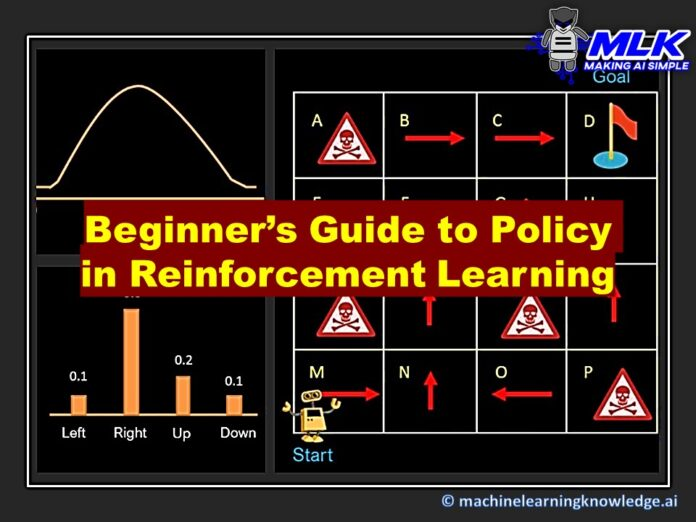# Beginner’s Guide to Policy in Reinforcement Learning## Introduction

Policy is the fundamental part of reinforcement learning that allows agents to make the right choices while interacting with the environment. In this article, we will understand what is policy in reinforcement learning and why it is important. We will then go through different types of policies – Deterministic Policy, Stochastic Policy, Categorical Policy, and Gaussian Policy.

## What is Policy in Reinforcement Learning

In a very simple term, a policy denoted by μ tells the agent what action to take at a particular state in the environment.

In the below animation, the agent has an action space of [Up, Down, Left, Right] and the arrows in each cell of the grid world are the policy that suggests to the agent what action to take in that state. For example, in cell ‘M’ the policy tells the agent to take right.

### Policy-Based Reinforcement Learning

At the very outset, the agent does not have a good policy in its hand that can yield maximum reward or helps him to reach its goal. The agent learns the good policy in an iterative process which is also known as the policy-based reinforcement learning method.

In this method, the policy is initialized randomly at the start. This means the agent takes random action at each state and then evaluates whether these actions lead to good or bad rewards.

Over several iterations, the agent starts learning the good policy that can produce positive rewards and can take him to its goal. At this point in time, we can say that the agent has learned an optimal policy.

### Optimal Policy

An optimal policy is a policy that yields good rewards for the agent and guides him to the goal. In our above animation, the policy shown is an optimal policy since it takes agents from the starting state M to the final goal state D.

Consider the state J, if the agent performs action Left or Right it will die with negative reward, if it goes Down it is moving away from its goal, hence the optimal policy tells it to move Up.

## Types of Policy in Reinforcement Learning

In reinforcement learning, the policy can be categorized as follows –

1. Deterministic Policy
2. Stochastic Policy

Let us do a deep dive into each of these policies.

### 1. Deterministic Policy

In a deterministic policy, there is only one particular action possible in a given state. When the agent reaches a given state, the deterministic policy tells it to perform a particular action always.

At any given time t, for a given state s, the deterministic policy μ tells the agent to perform an action a. It can be expressed as –

μ(s) = a

In the below gridworld example we have deterministic policy. For example,

μ(M) = Right , μ(J) = Up , μ(C) = Right

So whenever the agent visits state M, J, C it will perform action Right, Up, and Right respectively.

### 2. Stochastic Policy

In stochastic policy, it returns a probability distribution of multiple actions in the action space for a given state. It is in contrast with the deterministic policy which always mapped a given state to only one particular action.

So in this scenario, the agent may perform different actions each time it visits a particular state based on the probability distribution of actions returned by the stochastic policy.

At any given time t, for a given state s, the deterministic policy μ tells the agent to perform an action with a certain probability.

This can be expressed as μ(a|s)

In the below illustration, we can see that for each state the policy returns a probability distribution for the actions [Up, Down, Left, Right]. For example, in state J the stochastic policy returns [0.7, 0.1, 0.1, 0.1] which means that agent will move Up 70% of the time and will move Down, Left, or Right only 10% times.

### Types of Stochastic Policy

The stochastic policy can also be categorized into two types – i) Categorical Policy and ii) Gaussian Policy.

#### i) Categorical Policy

A stochastic policy is known as a Categorical Policy when the action space is discrete in nature. In this case, it uses a categorical probability distribution to select the actions from the discrete action space.

Our grid world example has discrete action space [Up, Down, Left, Right] and hence its stochastic policy can be classified as categorical policy.

#### ii) Gaussian Policy

A stochastic policy is known as Gaussian policy when the action space is continuous in nature. In this case, the policy uses Gaussian distribution over action space to select the action for a given state.

If we have an example where the agent has to drive a racing car and we consider the action space to be speed between 0mph to 200mph then this action space is continuous. Here the Gaussian policy selects the speed of the car from the Gaussian distribution over the action space of 0 to 200.